SSC (English Medium) Class 8Maharashtra State Board
Share

# Balbharati solutions for Class 8 Mathematics chapter 13 - Congruence of triangles

#### Balbharati Balbharati Class 8 Mathematics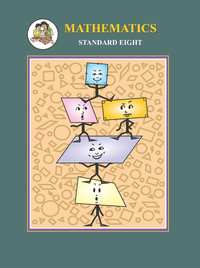## Chapter 13: Congruence of triangles

Practice Set 13.1OthersPractice Set 13.2

#### Chapter 13: Congruence of triangles Exercise Practice Set 13.1 solutions [Page 85]

Practice Set 13.1 | Q 1.1 | Page 85

In the following figure, parts bearing indentical marks are congruent. State the test and correspondence of vertices by which triangles in each pair are congruent.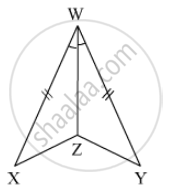Practice Set 13.1 | Q 1.2 | Page 85

In the following figures, parts bearing indentical marks are congruent. State the test and correspondence of vertices by which triangles in each pair are congruent.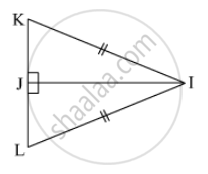Practice Set 13.1 | Q 1.3 | Page 85

In the following figures, parts bearing indentical marks are congruent. State the test and correspondence of vertices by which triangles en each pair are congruent.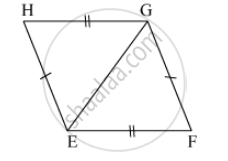Q 1.4 | Page 85

In the following figures, parts bearing indentical marks are congruent. State the test and correspondence of vertices by which triangles en each pair are congruent.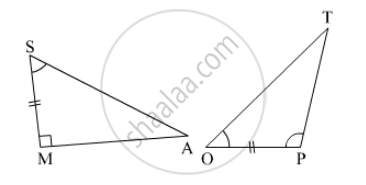Practice Set 13.1 | Q 1.5 | Page 85

In the following figures, parts bearing indentical marks are congruent. State the test and correspondence of vertices by which triangles en each pair are congruent.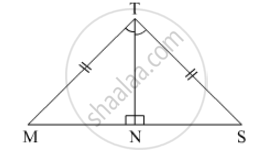#### Chapter 13: Congruence of triangles Exercise Practice Set 13.2 solutions [Page 87]

Practice Set 13.2 | Q 1.1 | Page 87

In the  triangle given below, parts shown by identical marks are congruent. State the test and the one to one correspondence of vertices by which triangles in each pair are congruent and remaining congruent parts.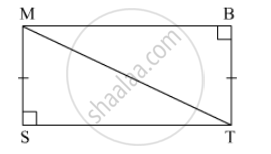Practice Set 13.2 | Q 1.2 | Page 87

In the triangle given below, parts shown by identical marks are congruent. State the test and the one to one correspondence of vertices by which triangles in each pair are congruent and remaining congruent parts.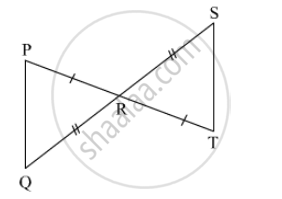Practice Set 13.2 | Q 1.3 | Page 87

In the triangle given below, parts shown by identical marks are congruent. State the test and the one to one correspondence of vertices by which triangles in each pair are congruent and remaining congruent parts.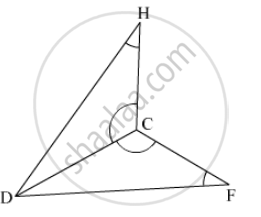Practice Set 13.2 | Q 2.1 | Page 87

In the adjacent figure, seg AD ≌ seg EC Which additional information is needed to show that ∆ ABD and ∆ EBC will be congruent by A-A-S test ?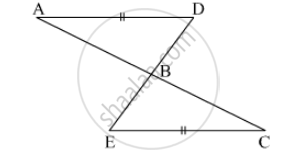## Chapter 13: Congruence of triangles

Practice Set 13.1OthersPractice Set 13.2

#### Balbharati Balbharati Class 8 Mathematics## Balbharati solutions for Class 8 Mathematics chapter 13 - Congruence of triangles

Balbharati solutions for Class 8 chapter 13 (Congruence of triangles) include all questions with solution and detail explanation. This will clear students doubts about any question and improve application skills while preparing for board exams. The detailed, step-by-step solutions will help you understand the concepts better and clear your confusions, if any. Shaalaa.com has the Maharashtra State Board Balbharati Class 8 Mathematics solutions in a manner that help students grasp basic concepts better and faster.

Further, we at Shaalaa.com provide such solutions so that students can prepare for written exams. Balbharati textbook solutions can be a core help for self-study and acts as a perfect self-help guidance for students.

Concepts covered in Class 8 Mathematics chapter 13 Congruence of triangles are Congruence of Triangles.

Using Balbharati Class 8 solutions Congruence of triangles exercise by students are an easy way to prepare for the exams, as they involve solutions arranged chapter-wise also page wise. The questions involved in Balbharati Solutions are important questions that can be asked in the final exam. Maximum students of Maharashtra State Board Class 8 prefer Balbharati Textbook Solutions to score more in exam.

Get the free view of chapter 13 Congruence of triangles Class 8 extra questions for and can use Shaalaa.com to keep it handy for your exam preparation

S